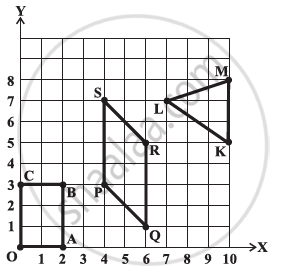Share

# Write the Coordinates of the Vertices of Each of These Adjoining Figures. - CBSE Class 8 - Mathematics

ConceptLinear Graphs - Location of a Point

#### Question

Write the coordinates of the vertices of each of these adjoining figures.#### Solution

The coordinates of the vertices in the given figure are as follows

O (0, 0), A (2, 0), B (2, 3), C (0, 3)

P (4, 3), Q (6, 1), R (6, 5), S (4, 7)

K (10, 5), L (7, 7), M (10, 8)

Is there an error in this question or solution?

#### APPEARS IN

NCERT Solution for Mathematics Textbook for Class 8 (2018 to Current)
Chapter 15: Introduction to Graphs
Ex. 15.20 | Q: 3 | Page no. 243
Solution Write the Coordinates of the Vertices of Each of These Adjoining Figures. Concept: Linear Graphs - Location of a Point.
S